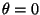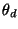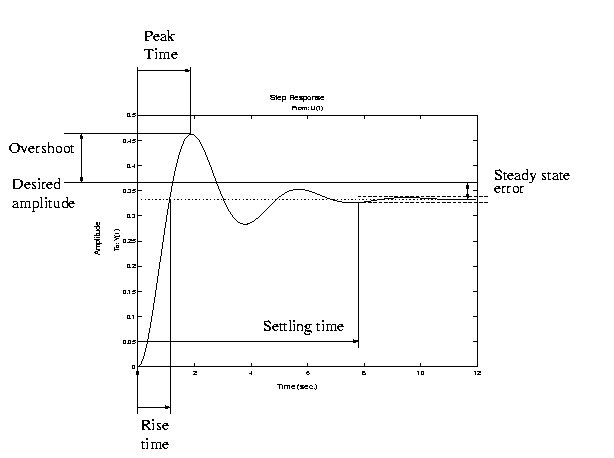AME 437: Control Systems Engineering Homework 1

 B. Goodwine Spring, 2003 Issued: January 17, 2002 Due: January 22, 2002

Consider the robot arm illustrated in the following figure, with a mass m and moment of inertia I.1.
Derive the equation of motion for the arm and linearize the equation about(we did this in class).
2.
Via numerical simulation of the linearized equations, (pick your own values for I, m, l and, and use any programming language and/or environment you want) verify the following rules of thumb'' for PID control in light of the definitions of rise time, peak time, overshoot, settling time and steady state error from the following figure.Submit plots indicating the response of the system as well as the gain values used.
(a)
For proportional control, i.e., kp > 0, kd = 0 and kI=0, the solutions are oscillatory, and increasing kp increases the frequency of oscillation (which decreases the rise time and peak time) but decreases the mean steady state error. The settling time is infinite.
(b)
If derivative control is added to the proportional controller, i.e., kp > 0, kd > 0 and kI=0, then
i.
for small kd the solutions are decaying oscillations;
ii.
increasing kd decreases the settling time;
iii.
increasing kd sufficiently eliminates the oscillatory behavior completely, resulting in an solution which exponentially decays to the final, steady state value;
iv.
increasing kp decreases the final steady state error;
v.
increasing kp decreases the rise time.
(c)
Adding integral control (PID control)
i.
eliminates the steady state error, even for small values of kp,
ii.
increasing kI generally increases the overshoot and settling time;
iii.
increasing kp decreases rise time, but may increase overshoot;
iv.
increasing kd increases damping and stability.

Last updated: January 17, 2003.
B. Goodwine (goodwine@controls.ame.nd.edu)